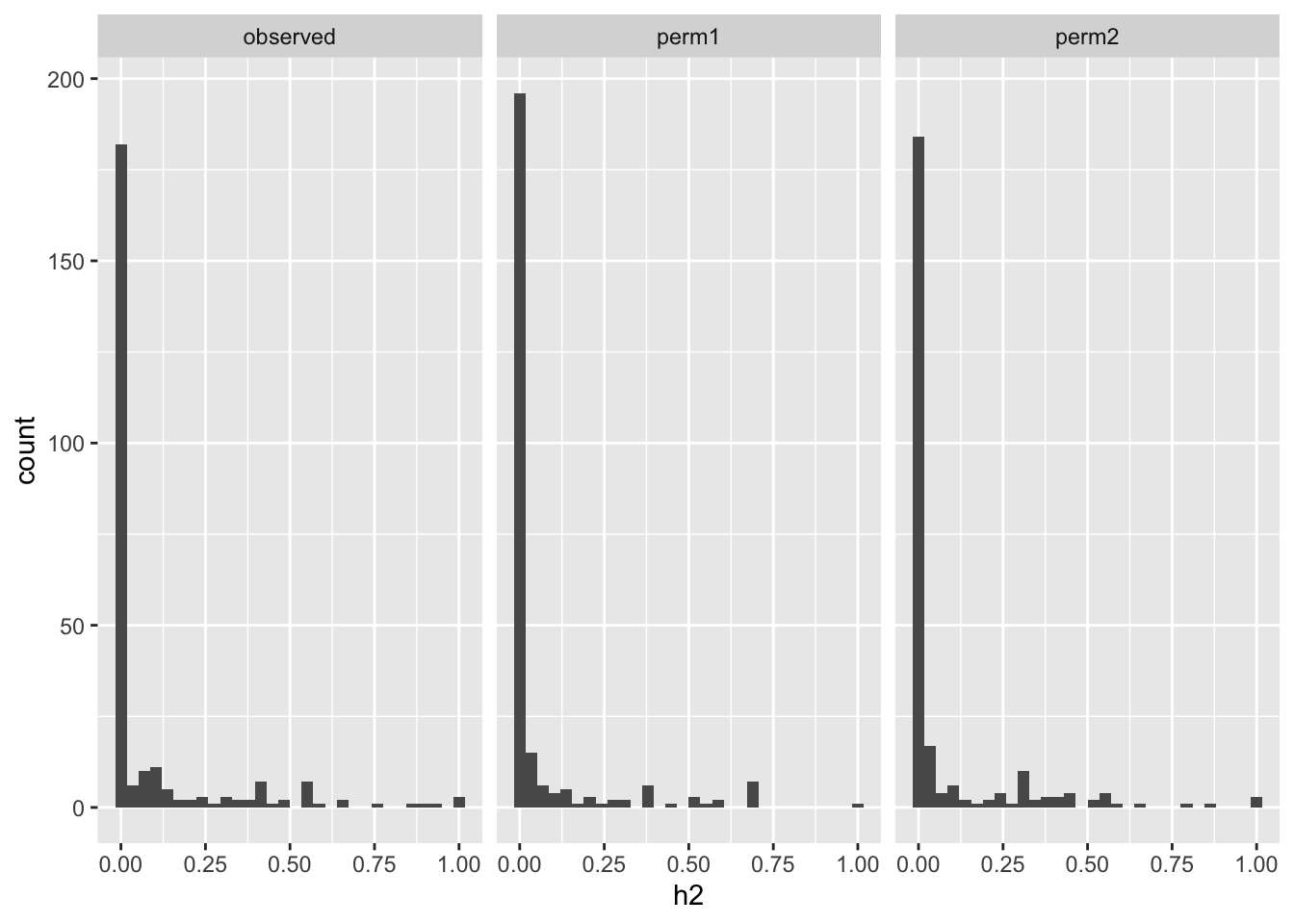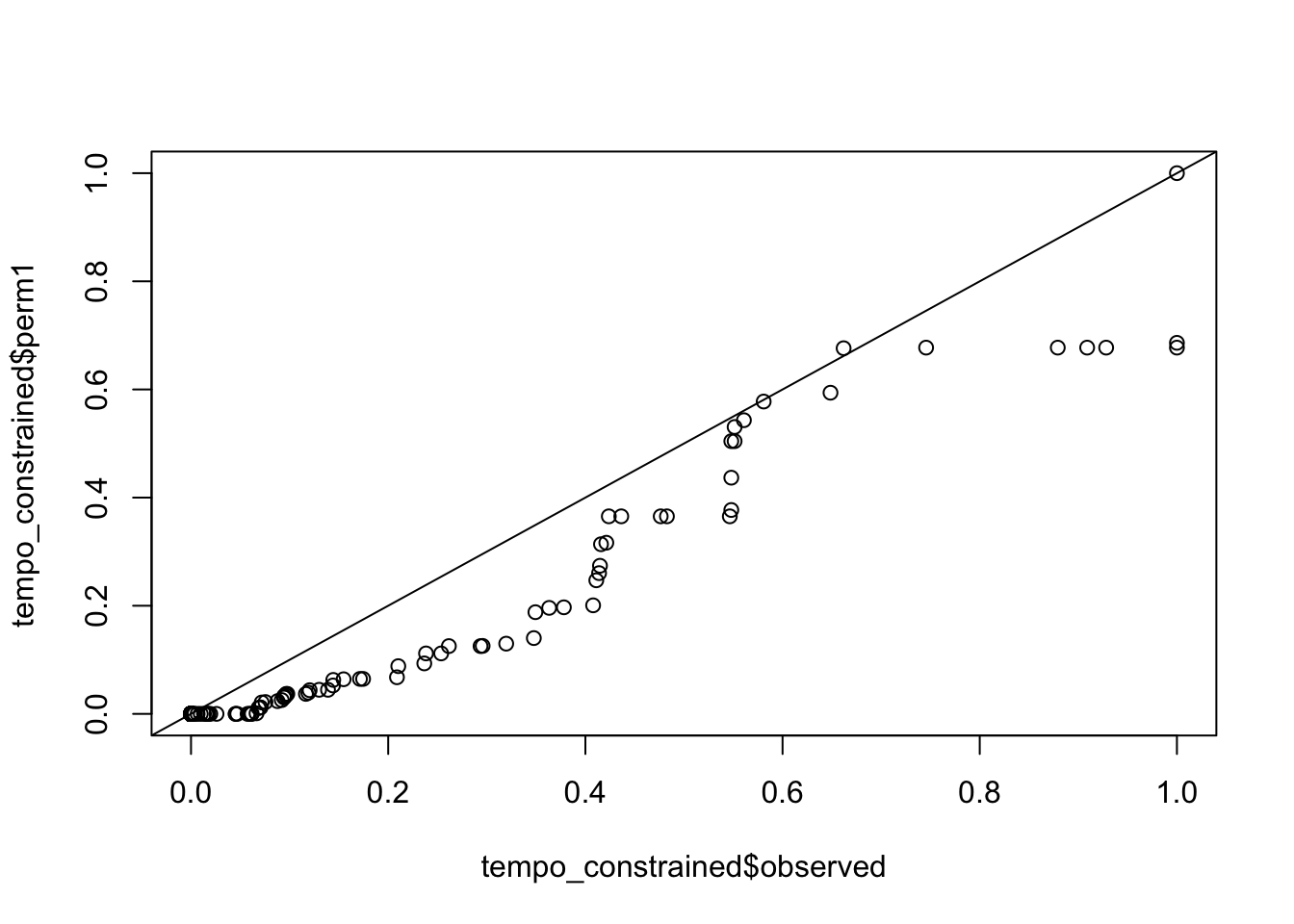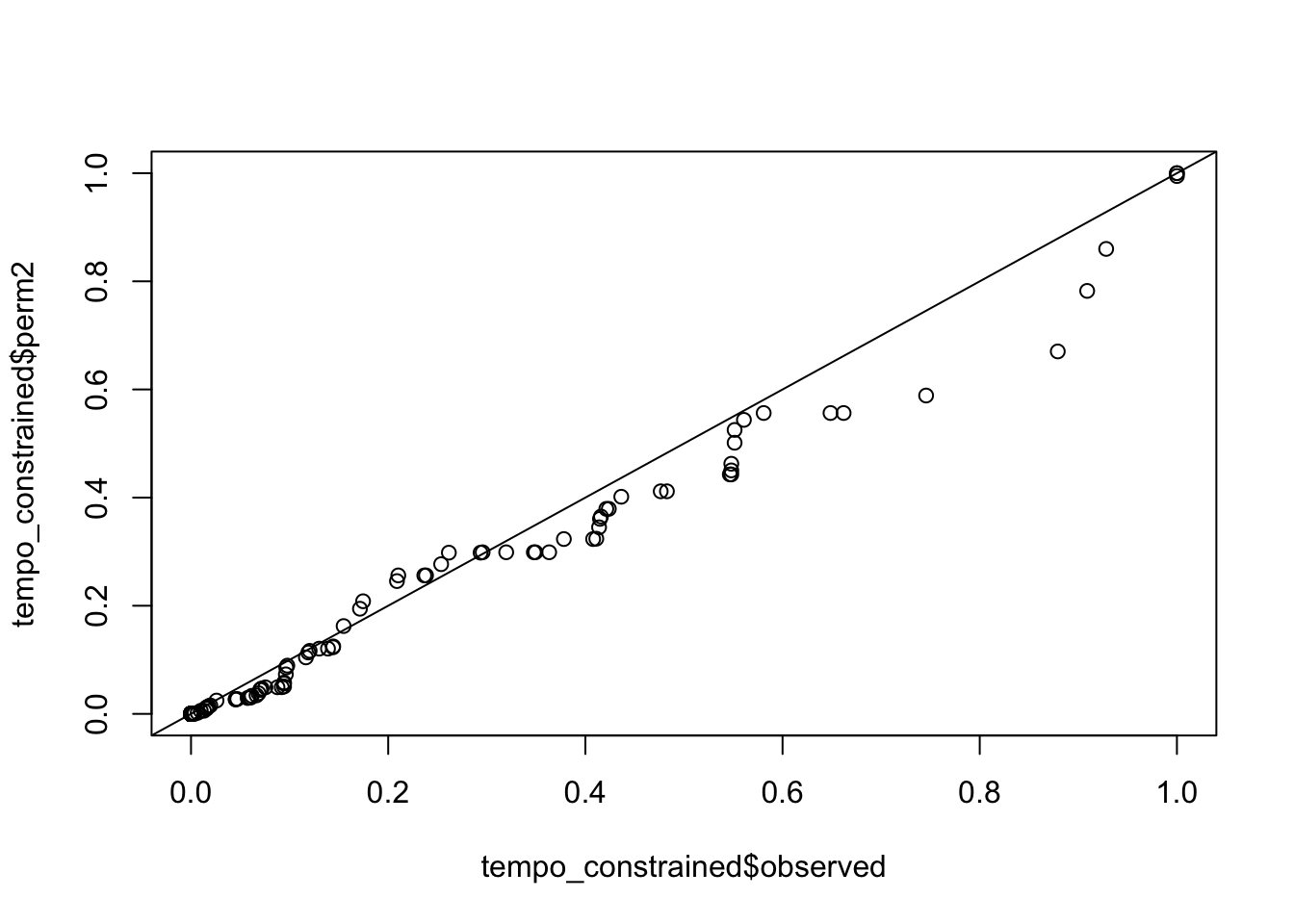# GTEx reaction rates h2 similar to permuted h2

Author

Haky Im

Published

November 2, 2020

We want to investigate whether reaction rates estimated with imat(?) are heritable. Reaction rates (binary variables) were estimated by D using GTEx gene expression data.

Initially, D generated reaction rates with brain cortex expression data from ~250 individuals.

`` ""``

Heritability of reaction rates was calculated with GCTA. Actual observed h2 and two permuted h2 values are compared next. Spoiler alert: no difference between actual and permuted values. :(

Show the code
``tempo = read_tsv(glue::glue("{DATA}/reaction-rates/df.GTEX.txt"))``
``````Rows: 256 Columns: 7
── Column specification ────────────────────────────────────────────────────────
Delimiter: "\t"
dbl (7): Phenotype, H2, Perm1, Perm2, H2 constrained, Perm constrained 1, Pe...

ℹ Use `spec()` to retrieve the full column specification for this data.
ℹ Specify the column types or set `show_col_types = FALSE` to quiet this message.``````
Show the code
``````tempo_constrained = tempo %>% select(pheno=Phenotype,observed=`H2 constrained`, perm1=`Perm constrained 1`, perm2=`Perm Constrained 2`)
tempo_constrained %>% pivot_longer(-pheno, names_to = "type", values_to="h2") %>% ggplot(aes(h2)) + geom_histogram() + facet_wrap(~type)``````
```stat_bin()` using `bins = 30`. Pick better value with `binwidth`.``qqplots comparing actual vs permuted h2

Show the code
``qqplot(tempo_constrained\$observed, tempo_constrained\$perm1); abline(0,1)``Show the code
``qqplot(tempo_constrained\$observed, tempo_constrained\$perm2); abline(0,1)``